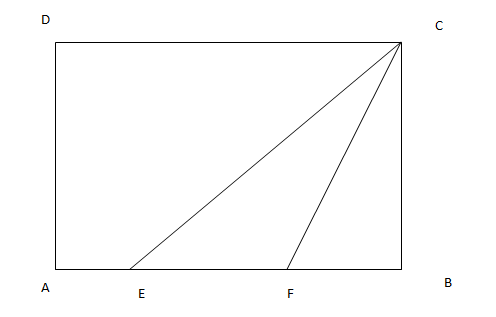Question 12

# In the given diagram, ABCD is a rectangle with AE = EF = FB. What is the ratio of the areas of CEF and that of the rectangle?Solution

Let the length of AB be 3X and the length of AD (and BC) be Y.

As the length of AB = 3X, AE = EF = FB =X

So, the area of the rectangle ABCD is length * breadth = 3X * Y = 3XY

The area of triangle CEF = 1/2 * base * height = 1/2 * EF * BC = 1/2*X*Y

So, required ratio = 1/2 : 3 = 1:6